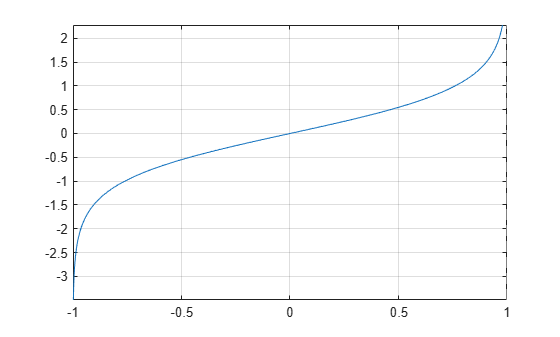# atanh

Symbolic inverse hyperbolic tangent function

## Syntax

``atanh(X)``

## Description

example

````atanh(X)` returns the inverse hyperbolic tangent function of `X`.```

## Examples

### Inverse Hyperbolic Tangent Function for Numeric and Symbolic Arguments

Depending on its arguments, `atanh` returns floating-point or exact symbolic results.

Compute the inverse hyperbolic tangent function for these numbers. Because these numbers are not symbolic objects, `atanh` returns floating-point results.

`A = atanh([-i, 0, 1/6, i/2, i, 2])`
```A = 0.0000 - 0.7854i 0.0000 + 0.0000i 0.1682 + 0.0000i... 0.0000 + 0.4636i 0.0000 + 0.7854i 0.5493 + 1.5708i```

Compute the inverse hyperbolic tangent function for the numbers converted to symbolic objects. For many symbolic (exact) numbers, `atanh` returns unresolved symbolic calls.

`symA = atanh(sym([-i, 0, 1/6, i/2, i, 2]))`
```symA = [ -(pi*1i)/4, 0, atanh(1/6), atanh(1i/2), (pi*1i)/4, atanh(2)]```

Use `vpa` to approximate symbolic results with floating-point numbers:

`vpa(symA)`
```ans = [ -0.78539816339744830961566084581988i,... 0,... 0.1682361183106064652522967051085,... 0.46364760900080611621425623146121i,... 0.78539816339744830961566084581988i,... 0.54930614433405484569762261846126 - 1.5707963267948966192313216916398i]```

### Plot Inverse Hyperbolic Tangent Function

Plot the inverse hyperbolic tangent function on the interval from -1 to 1.

```syms x fplot(atanh(x),[-1 1]) grid on```### Handle Expressions Containing Inverse Hyperbolic Tangent Function

Many functions, such as `diff`, `int`, `taylor`, and `rewrite`, can handle expressions containing `atanh`.

Find the first and second derivatives of the inverse hyperbolic tangent function:

```syms x diff(atanh(x), x) diff(atanh(x), x, x)```
```ans = -1/(x^2 - 1) ans = (2*x)/(x^2 - 1)^2```

Find the indefinite integral of the inverse hyperbolic tangent function:

`int(atanh(x), x)`
```ans = log(x^2 - 1)/2 + x*atanh(x)```

Find the Taylor series expansion of `atanh(x)`:

`taylor(atanh(x), x)`
```ans = x^5/5 + x^3/3 + x```

Rewrite the inverse hyperbolic tangent function in terms of the natural logarithm:

`rewrite(atanh(x), 'log')`
```ans = log(x + 1)/2 - log(1 - x)/2```

## Input Arguments

collapse all

Input, specified as a symbolic number, variable, expression, or function, or as a vector or matrix of symbolic numbers, variables, expressions, or functions.

## Version History

Introduced before R2006a